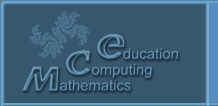Русский
 ! Вопросы и ответы

## Presentations

### The ray algebras in the models of quantum interactions

Koganov A.V.

Federal Science Center System Research Institute of Russian Academy of Sciences (FGU FNC NIISI RAN) (SRISA) Russia, Moscow, 117218, Nakhimovsky st., 36, corpus 1

In [1-2] the concept of ray algebra was introduced, which is a modification of Hypercomplex algebra. In these algebras, the negative unit is considered as one of the complex (ray) units. In this case, the dimension of the space of the Hypercomplex algebra is doubled, and the coefficients before the ray units are allowed only to be nonnegative. Accordingly, the multiplication tables of complex (ray) units are modified. The multiplication of vector ray numbers is determined by the distributivity to the multiplication of ray units. The base algebra becomes a nonnegative half-axis (ray) with the operations of addition (semigroup) and multiplication (group and ideal 0). There is no zero divisor in these algebras, but division is not possible for any pairs of numbers. All ray algebras are modular over both operations for the norm . There are automorphisms of algebras of radiation on Hypercomplex counterparts. Ray modifications of universal algebras with carriers on vector spaces are considered. In the most General case, these are measure algebras on a given Sigma algebra with an addition operation and special multivariate operations mapping the direct product of the measures of the operands into one measure on the same carrier. The resulting measure is normalized to the product of the norms of the operands (and for addition to the sum of the norms). This allows us to consider such algebras as a generalization of probability theory to the case of quantum superpositions in particle interaction models. Another feature of such algebras is the ambiguous solution of the Cauchy problem in differential equations. It is shown that the arising variations of the solution are analogous to the introduction of the virtual particle apparatus into Feynman diagrams. At the same time, they allow to avoid ultraviolet divergence and renormalization of the interaction model. Virtual particles are considered as pairs of annihilating real particles with total zero energy. The work was carried out on the topic of the state task NIR 0065-2019-0007.

References.

1. А. В. Коганов. Лучевые числа и алгебры. // Труды НИИСИ РАН, Т 9. № 1, Математическое и компьютерное моделирование сложных систем: теоретические и прикладные аспекты. 2019, Москва, ФГУ ФНЦ НИИСИ РАН, с. 98-107. ISSN 2225-7349.

2. 1. Коганов А. В. Лучевые алгебры и пространство-время. // Материалы 14-й Международной конференции «Финслеровы обобщения теории относительности» FERT-2018, 25-28.10.2018г.; ред. Павлов Д.Г., Панчелюга В.А.; Москва, РУДН, 2018 (ISBN 978-5-4465-1195-2), с.25-29.

3. И. Л. Кантор, Ф. С. Солодовников. Гиперкомплексные числа. М. «Наука», 1973

© 2004 Designed by Lyceum of Informational Technologies №1533